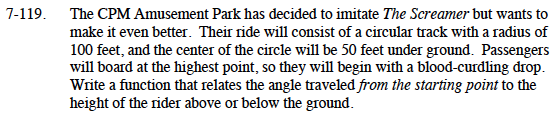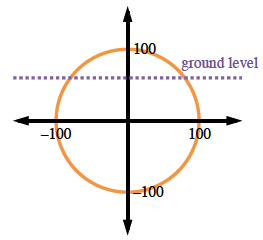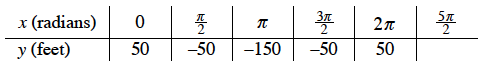Home > CCA2 > Chapter 7 > Lesson 7.2.1 > Problem7-119

7-119.

The CPM Amusement Park has decided to imitate The Screamer but wants to make it even better. Their ride will consist of a circular track with a radius of 100 feet, and the center of the circle will be 50 feet under ground. Passengers will board at the highest point, so they will begin with a blood-curdling drop. Write a function that relates the angle traveled from the starting point to the height of the rider above or below the ground. Homework Help ✎Begin by sketching a unit circle to model the situation.
When the passenger boards at 90° on the unit circle (but 0° traveled), they are
50 feet above ground.

$\text{When they have traveled }90\degree \left(\frac{\pi}{2}\text{ radians}\right),\text{ the passenger has moved to 50 feet}$

$\text{below ground, or the center point. }$

$\text{After traveling } 180\degree(\pi \text{ radians})\text{ they are 150 feet below ground. }$

$\text{After moving } 270\degree \left(\frac{\pi}{2} \text{ radians}\right), \text{they are back to } 50 \text{ feet above ground.}$

$\text{They return to } 50 \text{ feet above ground after turning a full circle, }360\degree(2\pi \text{ radians}).$Make a table of values then sketch the graph.Determine the parameters of the equation.
As with any periodic function, either y = sinx or y = cosx could be the parent graph for the equation.
Since we have started at a high point, we will use cosine as the parent.
Determine the horizontal and vertical shifts and the amplitude.

The horizontal shift (h) is 0. The vertical shift (k) is 50 feet down. The amplitude (a) is 100.
Substitute the parameters into the general equation, y = a · sin(xh) + k.
Use a graphing calculator to see that your equation matches your graph.

$y = 100\text{sin} \left(x + \frac{\pi}{2}\right) - 50\text{ or }y = 100 \text{cos}(x) - 50$